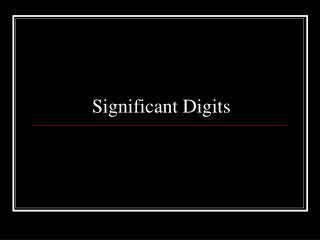DownloadDownload PresentationSignificant Digits

# Significant Digits

Download Presentation## Significant Digits

- - - - - - - - - - - - - - - - - - - - - - - - - - - E N D - - - - - - - - - - - - - - - - - - - - - - - - - - -
##### Presentation Transcript

1. Significant Digits

2. Objective • SWBAT • Determine the correct number of significant digits in a number.

3. Rule 1 • Digits other than zero are always significant. • Examples: 98765 5 sig. figs. 1.56 3 sig. figs.

4. Rule 2 • Any final zero or zeros used after the decimal point are significant. • Examples: 8.80 3 sig. figs. 15.000 5 sig. figs. Note: 100 has 1 sig fig. There is no decimal point.

5. Rule 3 • Zeros between two other significant digits are always significant. • Examples: 1001 4 sig. figs. 5.60023 6 sig. figs.

6. Rule 4 • Zeros used solely for spacing the decimal point are not significant. • Examples: 0.001 1 sig. fig. 0.011 2 sig. figs. 0.111 3 sig. figs.

7. Significant Digits in Addition and Subtraction • When quantities are being added or subtracted, the number of decimal places(not significant digits) in the answer should be the same as the least number of decimal places in any of the numbers being added or subtracted.

8. Addition Example • Example: • 5.67 J (two decimal places) 1.1 J (one decimal place) 0.9378 J (four decimal place) 7.7 J (one decimal place)

9. Addition and sig figs • 32.01 m5.325 m12 m • Added together, you will get 49.335 m, but the sum should be reported as '49' meters.

10. Significant Digits in Multiplication and Division • In a calculation involving multiplication, division the number of significant digits in an answer should equal the least number of significant digits in any one of the numbers being multiplied or divided.

11. Multiplication/Division and Sig Figs • If, for example, a density calculation is made in which 25.624 grams is divided by 25 mL, the density should be reported as 1.0 g/mL, not as 1.0000 g/mL or 1.000 g/mL. • Remember: least number of sig figs

12. When doing multi-step calculations, keep at least one more significant digit in intermediate results than needed in your final answer. • For instance, if a final answer requires two significant digits, then carry at least three significant digits in calculations. • If you round-off all your intermediate answers to only two digits, you are discarding the information contained in the third digit, and as a result the second digit in your final answer might be incorrect. (This phenomenon is known as "round-off error.")

13. The Two Greatest Sins Regarding Significant Digits 1. Writing more digits in an answer (intermediate or final) than justified by the number of digits in the data. 2. Rounding-off, say, to two digits in an intermediate answer, and then writing three digits in the final answer. http://www.physics.uoguelph.ca/tutorials/sig_fig/SIG_dig.htm

14. Web-site to Try • http://www.carlton.paschools.pa.sk.ca/chemical/Sigfigs/contents.htm